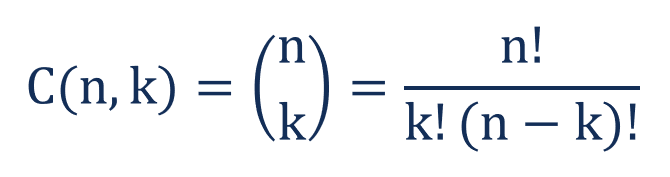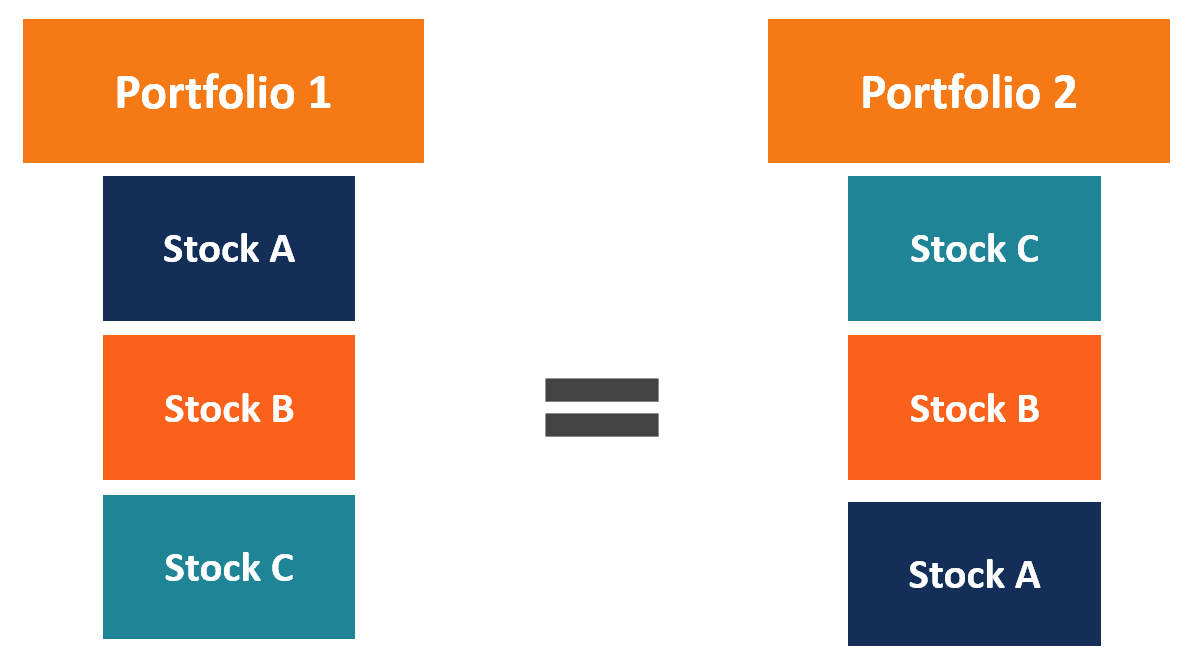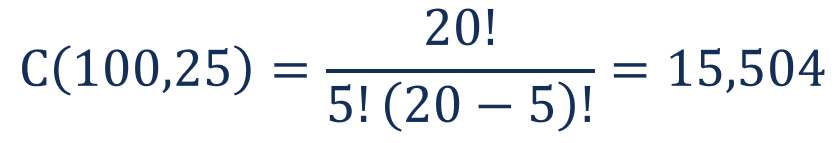# Combination

The number of possible arrangements in a collection of items where the order does not matter

## What is a Combination?

A combination is a mathematical technique that determines the number of possible arrangements in a collection of items where the order of the selection does not matter. In combinations, you can select the items in any order.Combinations can be confused with permutations. Nevertheless, in permutations, the order of the selected items is essential. For example, the arrangements ab and ba are equal in combinations (considered as one arrangement) while in permutations, the arrangements are different.

Combinations are studied in combinatorics but are also used in different disciplines, including mathematics and finance.

### Formula for Combination

Mathematically, the formula for determining the number of possible arrangements by selecting only a few objects from a set with no repetition is expressed in the following way:Where:

• n – the total number of elements in a set
• k – the number of selected objects (the order of the objects is not important)
• ! – factorial

Factorial (noted as “!”) is a product of all positive integers less or equal to the number preceding the factorial sign. For example, 3! = 1 x 2 x 3 = 6.

Note that the formula above can be used only when the objects from a set are selected without repetition.

### Example of Combination

You are a portfolio manager in a small hedge fund. You’ve decided to create a new fund that will attract risk-taking investors. The fund will include stocks of fast-growing companies that offer high growth potential. Your team of analysts identified the stocks of 20 companies that suit the profile.

Since it is a new fund, you’ve decided to include five stocks with equal weight in the initial portfolio, and after one year, you will review the performance of the portfolio and add new stocks if the performance will be successful. Currently, you want to identify the number of possible portfolios you can create from the stocks identified by your analysts.

The investment decision-making is an example of a combination problem. Since you are going to develop a portfolio in which all stocks will be of equal weights, the order of the selected stocks does not influence the portfolio. For example, the portfolio ABC and CBA would be equal to each other because of the similar weights (33.3% each) of each stock.Therefore, you can use the combination formula to calculate the number of possible arrangements:There are 15,504 possible portfolios of five stocks that can be created from 20 shortlisted stocks.